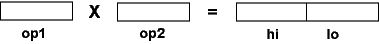No. In general, 64 bits are needed.

# MIPS Multiply Unit

The multiply unit of MIPS contains two 32-bit registers called hi and lo. These are not general purpose registers. When two 32-bit operands are multiplied, hi and lo hold the 64 bits of the result. Bits 32 through 63 are in hi and bits 0 through 31 are in lo.Here are the instructions that do this. The operands are contained in general-purpose registers.

```mult    s,t        # hilo <— \$s * \$t   (two's comp operands)
```
```multu   s,t        # hilo <— \$s * \$t   (unsigned operands)
```

There is a multiply instruction for unsigned operands, and a multiply instruction for signed operands (two's complement operands). Integer multiplication never causes a trap.

Note: with `add` and `addu`, both perform the same operation. The "u" means "don't trap overflow". With `mult` and `multu`, different operations are carried out. Neither instruction ever causes a trap.

### QUESTION 3:

Two small integers are multiplied. Where is the result?